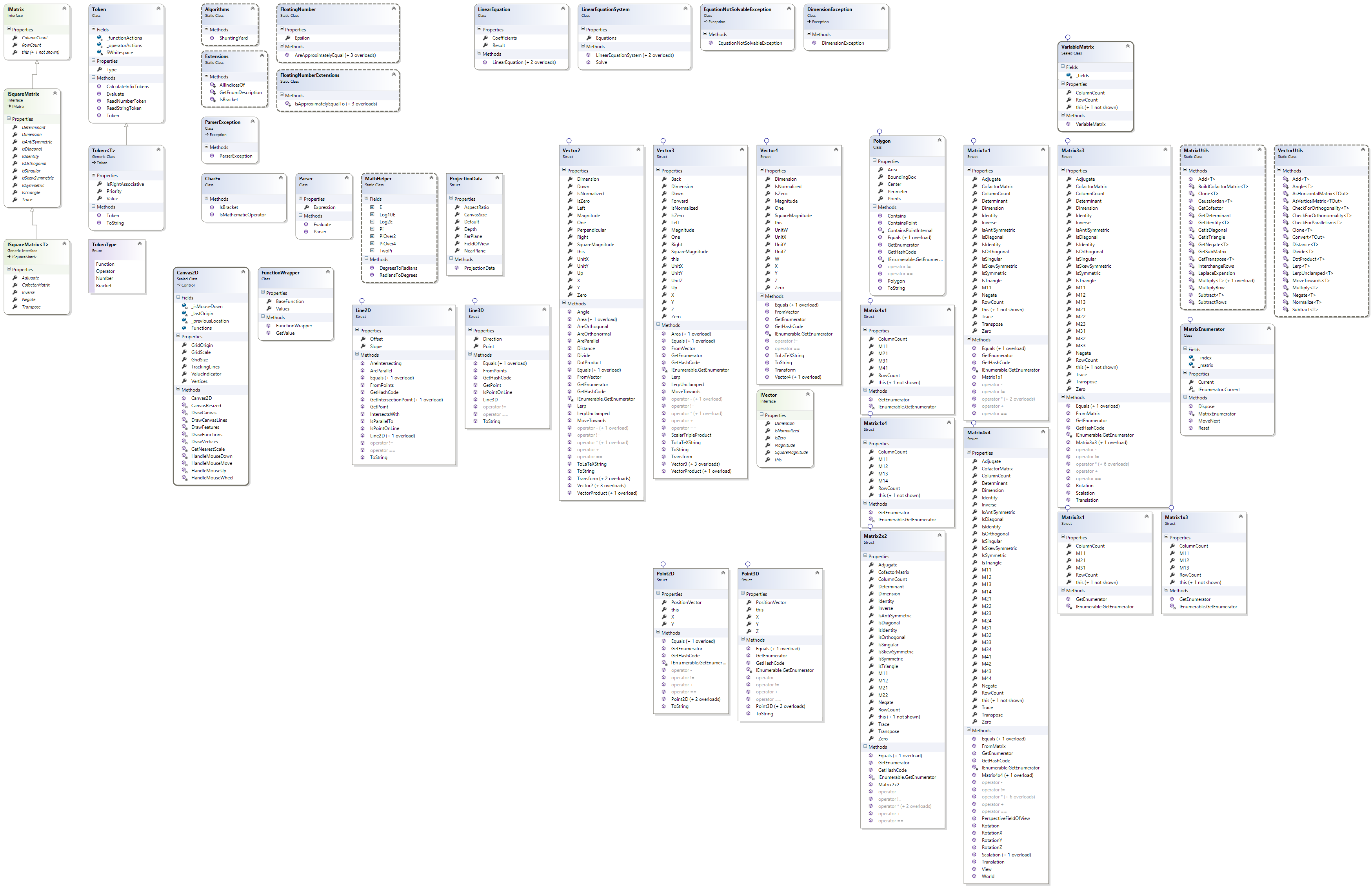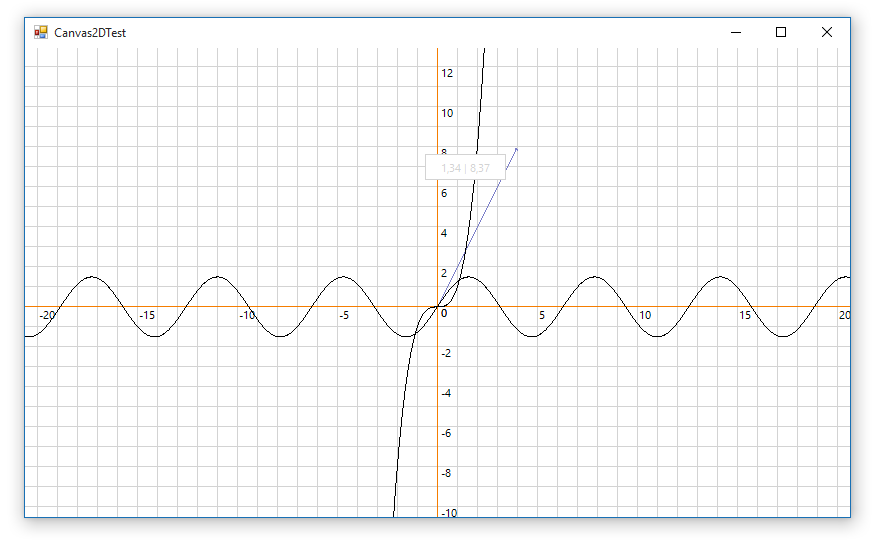# SharpMath

SharpMath is a C# math library supporting vectors, matrices, linear equation systems, expression parsing and soon more advanced analysis. Specials thanks go to NikxDa who wrote the Canvas2D control for representing vectors and functions graphically.

## Overview## 2D and 3D-Geometry

SharpMath offers many structs, such as points, vectors, lines and matrices. These are basically also used in games/computer graphics, except that they're a bit more extensive and sophisticated. Thus, the game engines provide some more flexibility and possibilites than SharpMath. Nevertheless, you can perform simple operations (such as rotating, scaling or translating objects defined through vertices) in space and project everything onto a two-dimensional surface.## Linear equation systems

SharpMath can solve linear equation systems using the Gauss-Jordan algorithm internally by representing the equations as matrices.

## Expression Parsing

SharpMath knows most of the functions, constants and operators used in computations. You can provide the parser with an input string containing your expression and it will return the result when calling the corresponding function. The flexibility of the parser allows you to add further operations. This feature may be used for calculators.## Canvas2D

SharpMath provides a two-dimensional canvas control for representing vectors and functions graphically in your application. It was written by NikxDa. A three-dimensional representation (e.g. for vectors, points and lines) will follow as well. Thanks to the `Geometry`-namespace, the implementation of this one should not be too hard as the projection onto a two-dimensional surface can be done using the integrated matrices.Get A Weekly Email With Trending Projects For These Topics
No Spam. Unsubscribe easily at any time.
C Sharp (275,773
Library (11,136
Dotnet (7,588
Parser (4,814
Math (3,551
Canvas (2,770
Calculator (1,901
Matrix (1,204
Functions (1,045
Vector (912
Vector (912
Geometry (838
Draw (371
Tokenizer (355
Polygon (334
Expression (272
Equation (161
Matrices (154
Linear (137
Related Projects